Next Article in Journal
Bio-Hydrogen Production from Pineapple Waste Extract by Anaerobic Mixed Cultures
Previous Article in Journal
A New Two-Stage Approach to Short Term Electrical Load Forecasting
Open AccessArticle

# Single Switched Capacitor Battery Balancing System Enhancements

1
Electrical Engineering and Energy Technology Department (ETEC), Vrije Universiteit Brussel, Pleinlaan 2, Brussel 1050, Belgium
2
Industrial Sciences and Technology (IWT), Erasmus University College, Nijverheidskaai 170, Brussel 1070, Belgium
*
Author to whom correspondence should be addressed.
Energies 2013, 6(4), 2149-2174; https://doi.org/10.3390/en6042149
Received: 31 December 2012 / Revised: 31 January 2013 / Accepted: 11 March 2013 / Published: 18 April 2013

## Abstract

Battery management systems (BMS) are a key element in electric vehicle energy storage systems. The BMS performs several functions concerning to the battery system, its key task being balancing the battery cells. Battery cell unbalancing hampers electric vehicles’ performance, with differing individual cell voltages decreasing the battery pack capacity and cell lifetime, leading to the eventual failure of the total battery system. Quite a lot of cell balancing topologies have been proposed, such as shunt resistor, shuttling capacitor, inductor/transformer based and DC energy converters. The shuttling capacitor balancing systems in particular have not been subject to much research efforts however, due to their perceived low balancing speed and high cost. This paper tries to fill this gap by briefly discussing the shuttling capacitor cell balancing topologies, focusing on the single switched capacitor (SSC) cell balancing and proposing a novel procedure to improve the SSC balancing system performance. This leads to a new control strategy for the SSC system that can decrease the balancing system size, cost, balancing time and that can improve the SSC balancing system efficiency.

## 1. Introduction

The BMS protects the battery system from damage, predicts and increases battery life, and maintains the battery system in an accurate and reliable operating condition. Battery pack cells’ imbalance is a major threat to the battery system life. The BMS performs several tasks such as measuring the system voltage, current and temperature, the cells’ state of charge (SoC), state of health (SoH), and remaining useful life (RUL) estimation, protecting the cells, thermal management, controlling the charge/discharge procedure, data acquisition, communication with on-board/off-board modules, monitoring, storing historical data and most importantly task is the cell balancing .
Without the balancing system, the individual cell voltages will drift apart over time. As well as, the usable capacity of the total battery pack will also decrease more quickly during operation that leads to fail the whole battery system [1,2].
Quite a lot of cell balancing/equalization methods have been proposed in [1,2,3,4,5,6,7,8,9,10,11,12,13,14,15,16,17,18,19,20,21] and reviewed in [1,2,3,4,5,6,7]. The battery balancing topologies can be divided into passive and active balancing; The passive battery balancing methods as proposed in [8,9] remove the excess energy from the fully charged cell(s) through a passive element, the resistor, until the charges match those of the lower cell(s) in the pack or a charge reference level. The resistor balancing can be either in fixed mode or switched resistor .
The active cell balancing methods remove the charges from higher energy cell(s) and deliver it to lower energy cell(s). Active cell balancing uses different topologies according to the active element for storing the energy such as capacitor and/or inductor component, as well as, the energy converters as [1,2,3,4,5,6,7,8,9,10,11,12,13,14,15,16,17,18,19,20,21]. The shuttling capacitor balancing has been subject to research [10,11,12,13,14,15,16,17,18,19]. Its simple control strategy and high efficiency are offset by a long equalization time and relatively high cost. Shuttling capacitor balancing methods can be classified into four configurations as shown in Figure 1: switched capacitor (SC), double-tiered switched capacitor (DTSC), single switched capacitor (SSC) and modularized switched capacitor (MSC).
Figure 1. Shuttling capacitor active cell balancing topologies.
Figure 1. Shuttling capacitor active cell balancing topologies.This paper focuses on the shuttling capacitor balancing topologies, approaching the methodology from different viewpoints, simulating different capacitor base balancing models using MATLAB/Simulink, and comparing between various shuttling capacitor balancing methods based on circuit configuration and simulation results. Finally, it proposes several improvements in the SSC balancing topology to overcome the switched capacitor long equalization time drawback, to decrease the system size and to solve the problem of the flat voltage curve of some types of lithium-ion batteries.

## 2. Shuttling Capacitor Cell Balancing Topologies

Shuttling capacitors cell balancing topologies, also known as “Charge Shuttling cells equalization” [10,11,12,13,14,15,16,17,18,19] basically utilize capacitors as external energy storage elements for shuttling the energy between the cells so as to perform the cells’ charge balancing. The capacitor shuttling can be categorized into four shuttling configurations: the basic switched capacitor, double-tiered switched capacitor, single switched capacitor and modularized switched capacitor topologies.

#### 2.1. Switched Capacitor

The switched capacitor (SC) illustrated in [1,2,3,4,5,10,11,12,13,18] is shown in Figure 2. It requires n − 1 capacitors and 2n bi-directional switches for balancing n cells. The SC control strategy is very simple because it has only two states, shuttling between the whole cells sequentially, moving the switches frequently from the upper position to the lower position and again to the upper one with a small rest period between each transition and so on.
In addition, it does not need any intelligent control strategy, it can work in both charging and discharging modes (at light load currents) and it operates with high efficiency. The disadvantages of the switched capacitor topology are its relatively long equalization time and its higher cost compared with the switched shunt resistor passive balancing method.
Figure 2. Switched capacitor cell balancing topology.
Figure 2. Switched capacitor cell balancing topology.#### 2.2. Double-Tiered Switched Capacitor

The DTSC balancing method given by [14,15,16,18] is a derivation of the switched capacitor method. The difference is that, the DTSC uses two capacitor tiers for energy shuttling as shown Figure 3. As illustrated it needs n capacitors and 2n bi-directional switches for balancing n cells.
Figure 3. Double-tiered switched capacitor cell balancing topology.
Figure 3. Double-tiered switched capacitor cell balancing topology.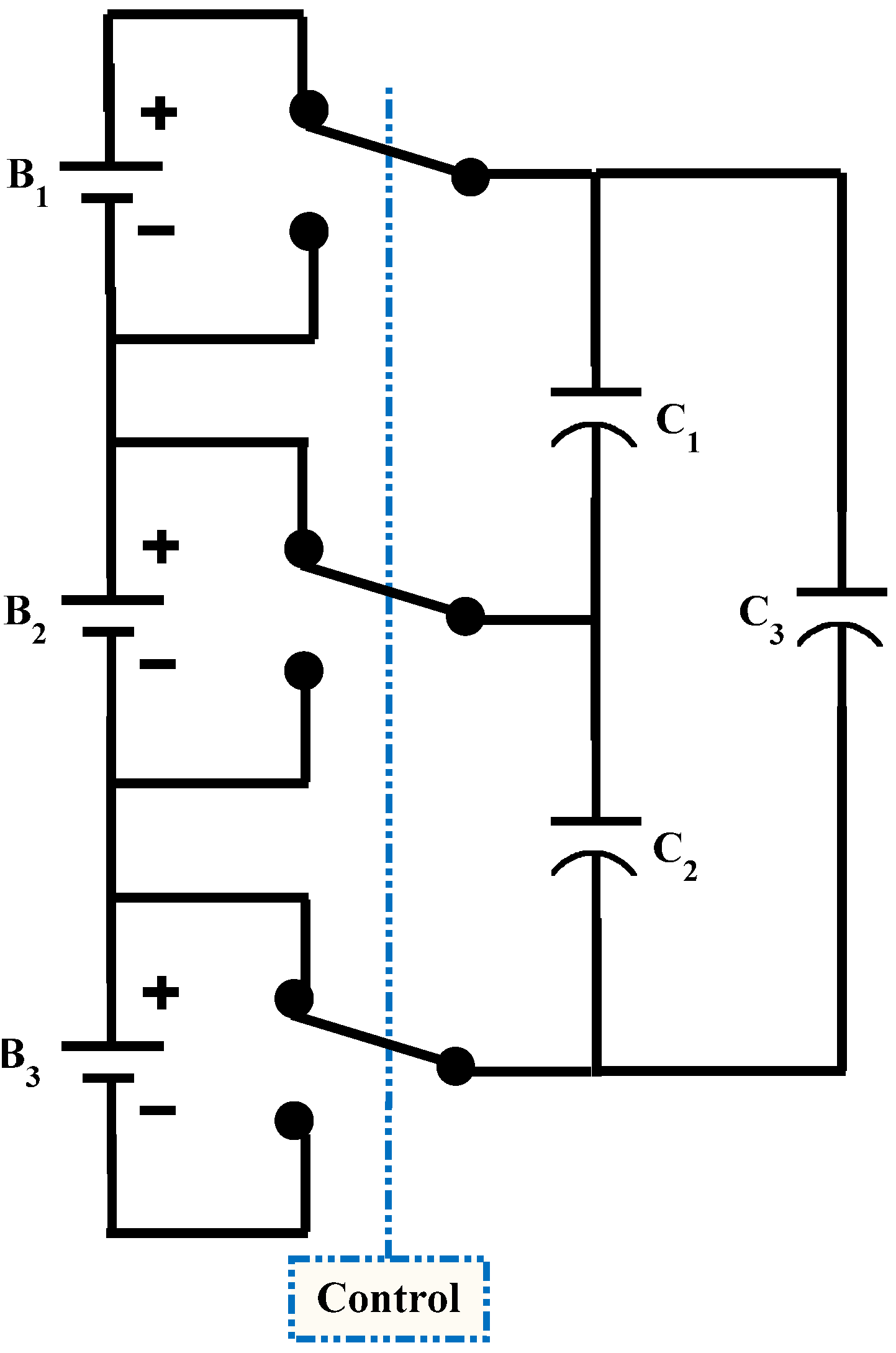Having more tiers means more paths between batteries, and thus less impedance for the transport of charge over a particular distance across the pack . The advantage of the double-tiered switched capacitor over the switched capacitor method is that the second capacitor tier reduces the balancing time to more than half. In addition, it can operate in both recharging and discharging with high efficiency.

#### 2.3. Single Switched Capacitor

Another derivation of the SC topology, the single switched capacitor (SSC) topology [1,2,4,5,17,18,19] makes use of only one capacitor as shown in Figure 4. The SSC needs a single capacitor and n + 5 bi-directional switches to balance n cells, making it more cost-efficient than SC and DTSC.
Figure 4. Single switched capacitor cell balancing topology.
Figure 4. Single switched capacitor cell balancing topology.A relatively simple control strategy is always used, where the controller selects the high and low energy cells and controls the corresponding switches for shuttling the energy between them. However, more advanced control strategies can be used for increasing the balancing speed, which will be discussed later in the proposed control strategy. Generally the capacitor balancing used for balance more than 4 cells because of its size and cost. For sure if the capacitor is used for balancing 4 cells or less (n ≠ 1) the SC will have fewer switches as mentioned in Table 1. But for more than 5 cells the SSC will have less switches than the SC system.
Table 1. SC vs. SSC balancing system switches according to the number of cells.
Table 1. SC vs. SSC balancing system switches according to the number of cells.
No. of cellsSC switches (2n)SSC Switches (n + 5)
247
368
489
51010
61211
81613
163221

#### 2.4. Modularized Switched Capacitor

Modularized switched capacitor (MSC) is another topology utilizing the shuttling capacitor method. It is based on battery pack modularization  as shown in Figure 5, dividing the battery pack into groups or modules.
Figure 5. Modularized switched capacitor cell balancing .
Figure 5. Modularized switched capacitor cell balancing .Inside each module a separate equalization system deals with individual cells, whileas another equalization system operates on module level. This reduces the balancing time but increases the number of capacitors and the switches (n − 1 capacitor low voltage, 1 capacitor high voltage and 2n + 4 bi-directional switches to balance n cells). The main drawback of the MSC is that, increasing the number of switches and capacitors will increase the losses and balancing system cost significantly.

## 3. Shuttling Capacitors Balancing Topologies Simulation

MATLAB/Simulink has become the leading software for modeling and simulating dynamic systems. In this paper it is used for simulating the shuttling capacitor balancing topologies. As a first step, the individual battery cells are simulated. Four lithium polymer (Li-Po) batteries have been tested and their models’ parameters estimated according to [22,23]. The chosen battery model was proposed by the “Extended Partnership for a New Generation of Vehicles (EPNGV)” . This battery model features SoC, SoH and cycle number prediction, variable parameters in function of SoC, temperature and cycle number with a real parameters variation between the pack cells.
The shuttling capacitors battery balancing methods (SC, DTSC and SSC) have been simulated using Simulink with the suitable control systems and no load current. Four 12 Ah lithium-ion cells are used for the simulation comparison, with initial SoCs of 87%, 85%, 78% and 76% respectively, with a 2%, 7% and 2% state of charge (SoC) difference between the two neighboring cells, means that the higher SoC difference is 11% or 1.32 Ah. As well as, the variation in the batteries models’ parameters have been utilized. The balancing considered to be occurred if the maximum SoC difference between the cells is 5% (0.6 Ah).
MOSFET switches are used in the simulation. They have an internal on-resistor, diode forward resistor of 15, 10 milliohm respectively and the diode forward voltage is 0.05 V. The MOSFETs snubber circuits are 50 KΩ and 250 nF.
The capacitors are used for SC and DTSC have a capacity of 33 mF with ESR of 25 mΩ. For the SSC the capacitor used has a capacity of 100 mF with ESR of 20 mΩ. The switching frequency (F) will be 200 Hz with a duty cycle (D) of 45%.
Figure 6, Figure 7 and Figure 8 illustrate the shuttling capacitors topologies simulations results such as: the cells’ voltages, SoCs, and currents, as well as, the cells’ energy content. In some cases one of the capacitor’s voltage and current will be shown as well.
The energy losses during the balancing process in Wh can be calculated from the subtraction of the cells’ energies summation at start (beginning of the balancing process) and at the end (cell balancing has occurred).
Switched capacitor balancing simulation results is shown in Figure 6, double-tiered switched capacitor balancing simulation results are shown in Figure 7, and the single switched capacitor simulation results are illustrated in Figure 8.
Figure 6 shows the SC balancing topology simulation results. It is clear that for an 11% SoC difference between the higher and lower cells, a very long time is needed for complete balancing. In addition, the low balancing current during the final balancing phase (due to the lower voltage differences) leads to a further increase of the balancing time. It took long equalization time about 4 hours and even more. However, energy losses (about 125 mWh during balancing) are much lower than with the switched resistor passive balancing system.
Figure 7 presents the DTSC balancing topology simulation results. As in the SC balancing system the balancing currents decrease along the balancing process. The balancing time is lower than for the SC because of the extra capacitor tier but it is still relatively long at about 1.7 hours. Just like the SC, the DTSC has high efficiency (about 105 mWh loss during balancing).
Figure 8 illustrates the single switched capacitor balancing topology simulation results. The low currents during the final balancing phase lead to a balancing time nearly of 3.4 hours, which can be decreased through the use of a more intelligent control strategy. The SSC has more efficiency than the SC and the DTSC (during balancing it loss about 110 mWh).
As an initial conclusion from the previous circuits and the simulation results it is clear that;
• The SSC has only one capacitor and less switches while the MSC balancing method utilizes more capacitors and switches than the traditional SC and DTSC balancing methods.
• Both SC and DTSC have a straightforward control strategy, on the contrary, the SSC and MSC need a relatively complex control.
• The SC and DTSC methods with a simple control strategy have a long final balancing time and have a great problem that, when the SoC difference between the cells is small, as well as, the voltage difference, the equalization current becomes smaller. That will increase the equalization time significantly.
• Compared to the SC method, the DTSC has one more capacitor, however it decreases the balancing speed up to 42%, some times more, of the normal SC.
Figure 6. Switched capacitor balancing simulation results (top to bottom) the cells and capacitors voltages, cells SoCs, currents, energies, and summation of the cells energy.
Figure 6. Switched capacitor balancing simulation results (top to bottom) the cells and capacitors voltages, cells SoCs, currents, energies, and summation of the cells energy.Figure 7. Double-tiered switched capacitor simulation (top to bottom) cells and capacitor C1 (between cells 1&2) voltages, cells SoCs, currents, cells energies, and summation of the cells energy.
Figure 7. Double-tiered switched capacitor simulation (top to bottom) cells and capacitor C1 (between cells 1&2) voltages, cells SoCs, currents, cells energies, and summation of the cells energy.Figure 8. Single switched capacitor balancing simulation (top to bottom) the cells and the capacitor voltages, cells SoCs, currents, energies, and summation of the cells energy.
Figure 8. Single switched capacitor balancing simulation (top to bottom) the cells and the capacitor voltages, cells SoCs, currents, energies, and summation of the cells energy.## 4. Single Switched Capacitor Control Strategy

Single switched capacitor cell balancing can optimize its performance by using a more efficient control strategy, reducing the capacitor size, the system cost, and the balancing time. The idea is to maximize the shuttling energy transfers between the cells, and minimizing the capacitor size and the balancing time. This will be done by intelligently controlling the switches after extracting the energy cost function(s).
Conventionally the control of the SSC is based on selecting the high voltage, cell and low voltage cell and shuttling the energy from the higher cell into the lower one. The switch control can be classically performed using a fixed frequency (F) and duty cycle (D) that controls the switched capacitor equivalent resistance Requ, presented in Equation (1) as a general case [25,26]. For normal duty cycle control, typically T is fixed, and D1 and D2 are both set close to 50%. The resistances are nearly equal as well, so that τ1 and τ2 are nearly equal to (ESR + RCell) * C. In this method a low equivalent resistance is paramount for effective equalization given by  as shown in Equation (1):This will not be very effectively when the voltage difference between the cells is small, when the equalization current becomes smaller and the equalization time will increase significantly.

#### 4.1. SSC Proposed Control Strategy

The “high charge cell–capacitor–low charge cell” energy shuttling is a function of the capacitor value (C), switching frequency (F), series equivalent resistor (RSeq), voltage different between the unbalanced cells (Vdiff) and finally the duty cycle, on-period, (D). The proposed SSC balancing strategy will be based on adapting these factors as described later.
Starting from the capacitor voltage during charging with an initial voltage Vi and final voltage Vf as shown in the first part of Figure 9, it can be expressed as in Equation (2), and the corresponding capacitor current can be formulated as Equation (3). These equations will be used for extracting the switched capacitor shuttling energy between the two cells.
Figure 9. Capacitor voltage during shuttling between two cells.
Figure 9. Capacitor voltage during shuttling between two cells.There are some assumptions concerning the transferred energy function. Firstly, the capacitor will switch from the lower charge cell with a voltage of Vi and connect to the higher charge cell with Vf voltage. Secondly, the cells’ internal resistance is equal, so that the series equivalent resistor (RSeq) of the switched capacitor circuit will be the sum of the capacitor ESR and one cell internal resistor and the time constant τ is equal to (ESR + Rcell)*C or RSeq.C. Thirdly, the SSC energy is calculated during one charging period only, so that time integration will be from zero to one duty cycle DT.
Equation (4) gives the energy transferred from the higher charge cell to the capacitor during the period D1T or DT in Ws/pulse. This energy is a function of the capacitor value C, switching frequency F, cells voltages, voltages difference Vdiff, series equivalent resistor RSeq, and duty cycle D. The number of pulses (e.g., in one hour) should be added into the charging energy (Ws/pulse) in Equation (4) to be in Wh/h as in Equation (5). This is shown in Figure 10: the top part shows the transferred energy in Ws/pulse and it has a “reverse” relation with the switching frequency. While the bottom part shows the transferred energy in Wh/h and it has a “direct” relation with the switching frequency.
Figure 10. Capacitor charging energy as a function of the switching frequency, (a) energy in Ws/pulse; (b) energy in Wh/h.
Figure 10. Capacitor charging energy as a function of the switching frequency, (a) energy in Ws/pulse; (b) energy in Wh/h.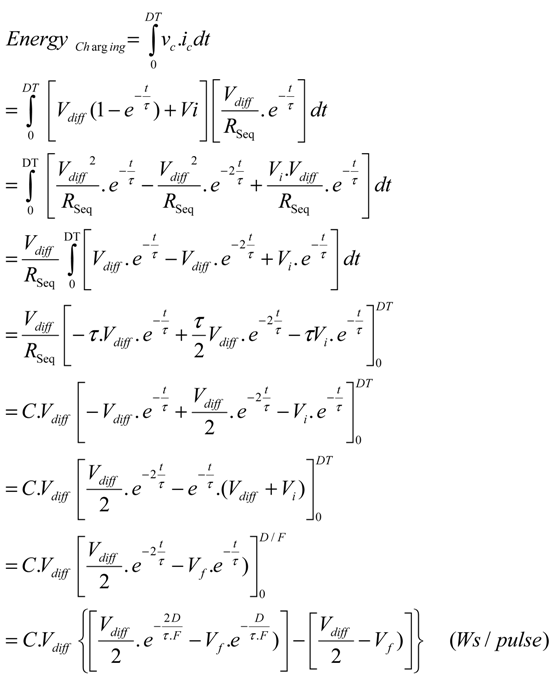The capacitor transferred energy during one discharge pulse can be calculated from the capacitor discharge voltage and current as given in Equation (6):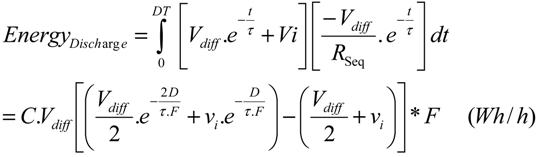Figure 11, Figure 12, Figure 13 and Figure 14 show the different relations between the charging energy as in Equation (5) and its variables C, Vdiff, RSeq and D, respectively.
Figure 11. Capacitor charging energy as a function of the capacitor value.
Figure 11. Capacitor charging energy as a function of the capacitor value.Figure 12. Capacitor charging energy as a function of the cells voltage difference.
Figure 12. Capacitor charging energy as a function of the cells voltage difference.Figure 13. Capacitor charging energy as a function of the series equivalent resistor RSeq.
Figure 13. Capacitor charging energy as a function of the series equivalent resistor RSeq.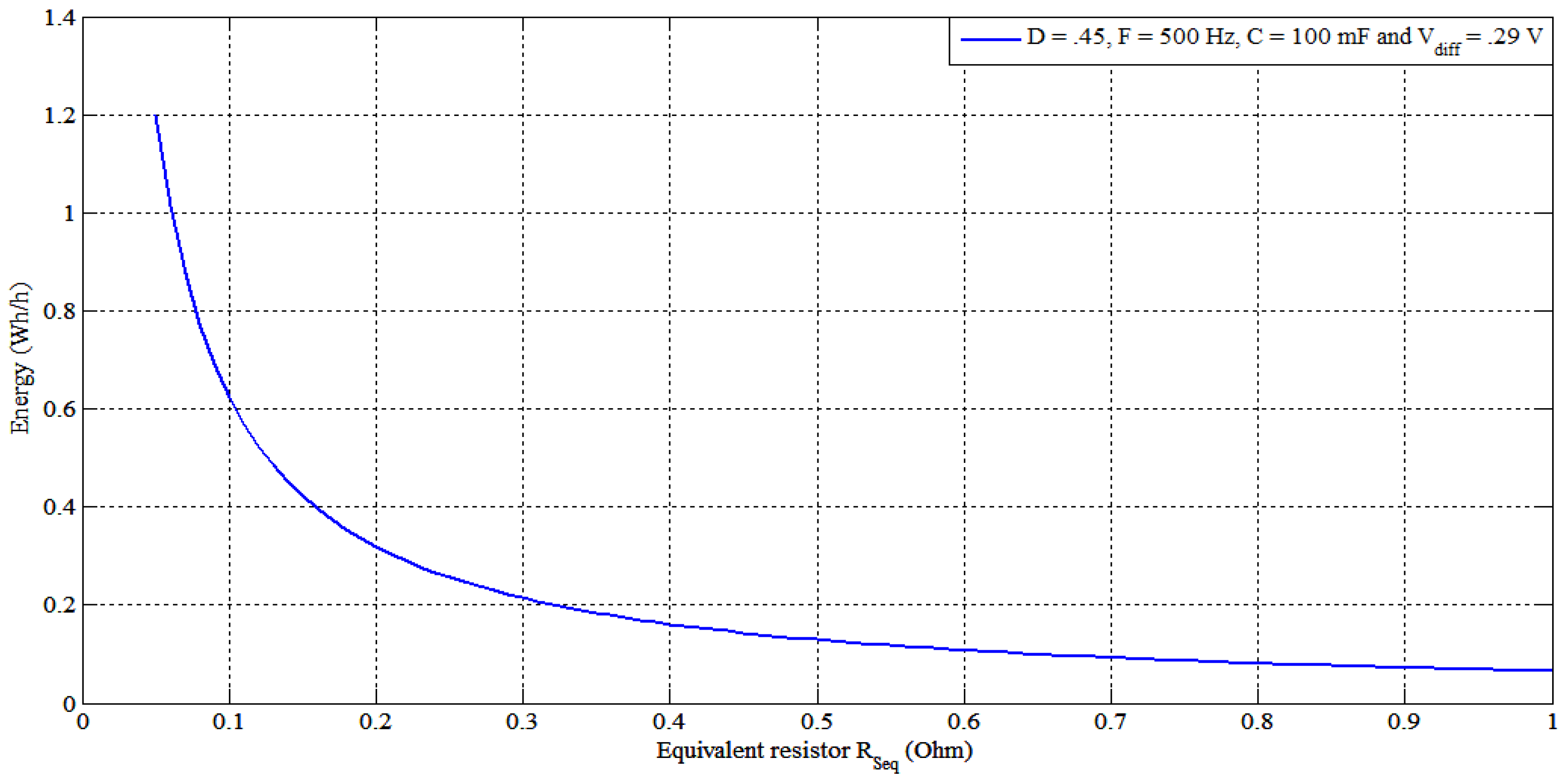Figure 14. Capacitor charging energy as a function of duty cycle D.
Figure 14. Capacitor charging energy as a function of duty cycle D.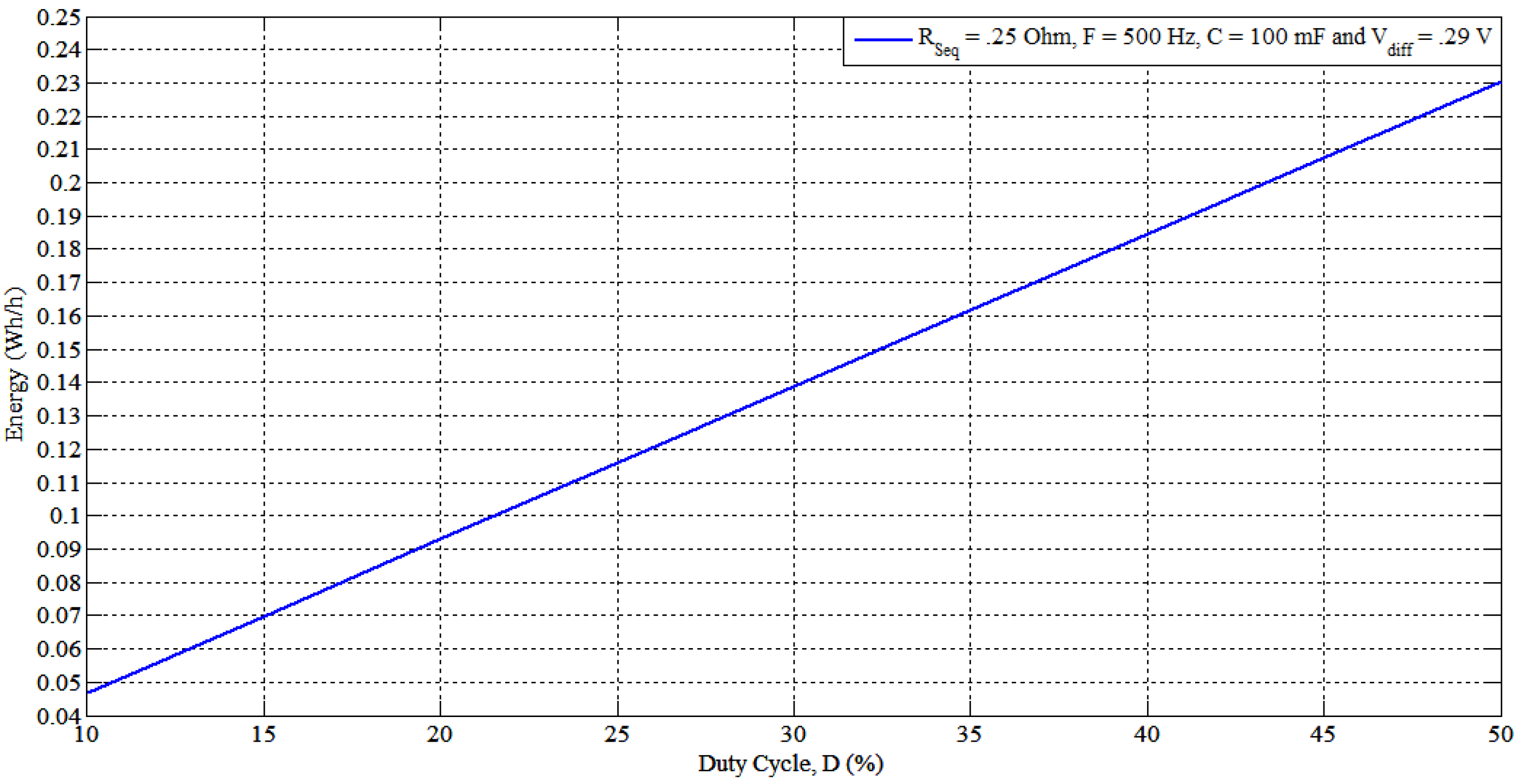As shown in the Figure 10, Figure 11, Figure 12, Figure 13 and Figure 14 and Equation (5) the transferred charging energy has different relations with C, F, Vdiff, RSeq and D. The aim of the proposed SSC cell balancing control strategy is to maximize this energy transfer with respect to these parameters (C, F, Vdiff, RSeq and D), as well as, to keep this energy as high as possible within any conditions e.g., variation of one parameter such as Vdiff decreasing during the balancing period.

#### 4.2. SSC Strategy Proceeding

The proposed control strategy for the SSC balancing can be summarized in the following steps:
• Extracting the function of the transferred energy between the cells and the capacitor [see Equation (5)]. This function can be easily maximized for transferred energy, but has to take into account the five premonition variables (C, F, Vdiff, RSeq and D).
• Selecting the optimal capacitor value (minimum) by maximizing the energy function with respect to the capacitor value at different switching frequencies. The transferred energy will be a function of (C, F), with any arbitrary Vdiff and D because the latter two parameters will vary during balancing periods.
• After select the capacitor value with the given ESR, maximizing the energy transfer with the calculated C and RSeq values as a function of (F, D) at different values of Vdiff.
• Dividing the balancing period into zones according to voltage difference Vdiff, and decide the required maximum current allowed through the capacitor and the corresponding equivalent resistor RSeq value. This resistor value allows to select a D value and the allowable switching frequency range as illustrated in Figure 15.
• By knowing the higher and lower cell voltage, applying the corresponding F and D along the balancing time according to the cell voltages to get the maximum energy transfer.
Figure 15. SSC resistor RSeq as a function the switching frequency and the duty cycle D.
Figure 15. SSC resistor RSeq as a function the switching frequency and the duty cycle D.6.
The big challenge of using the shuttling capacitor topologies with Li-ion batteries is their nearly flat voltage during the discharge curves shown in Figure 16. This is particularly the case for lithium iron phosphate batteries. A 10 mV difference is nearly equal 10% SoC in the flat region for the Li-ion batteries compared with 20 mV per 10% SoC for the lead-acid batteries. In addition, one has to consider the voltage drops in the switches, so the capacitor balancing will have difficulties to equalize the cells with a very small voltage difference.
Figure 16. Different Li-Ions chemistry OCV-SoC curve.
Figure 16. Different Li-Ions chemistry OCV-SoC curve.The solution of this problem is done by boosting the capacitor voltage in the period between the charging and discharging pulses (at the rest period) to increase the capacitor voltage a little higher than the high charge cell before connecting the capacitor to the low charge cell.
The capacitor voltage boosting can be achieved by using one small isolated dc-dc converter (MEE3S1205SC) as shown in Figure 17. The capacitor voltage at the small boosting pulse is controlled using a microcontroller, which controls the small pulse width to adjust the capacitor boosting voltage.
Figure 17. SSC voltage boosting topology using small isolated dc-dc converter.
Figure 17. SSC voltage boosting topology using small isolated dc-dc converter.Figure 18 shows the capacitor voltage experimentally when applying the boosting technique; at the first period, the capacitor is connected to the higher cell (capacitor charging) then it is connecting to the small dc-dc converter (capacitor boosting) and finally the capacitor is connected to the lower cell (capacitor discharging) with inter-between rest periods, and so on.
Figure 18. Experimental capacitor voltage charging-boost-discharging.
Figure 18. Experimental capacitor voltage charging-boost-discharging.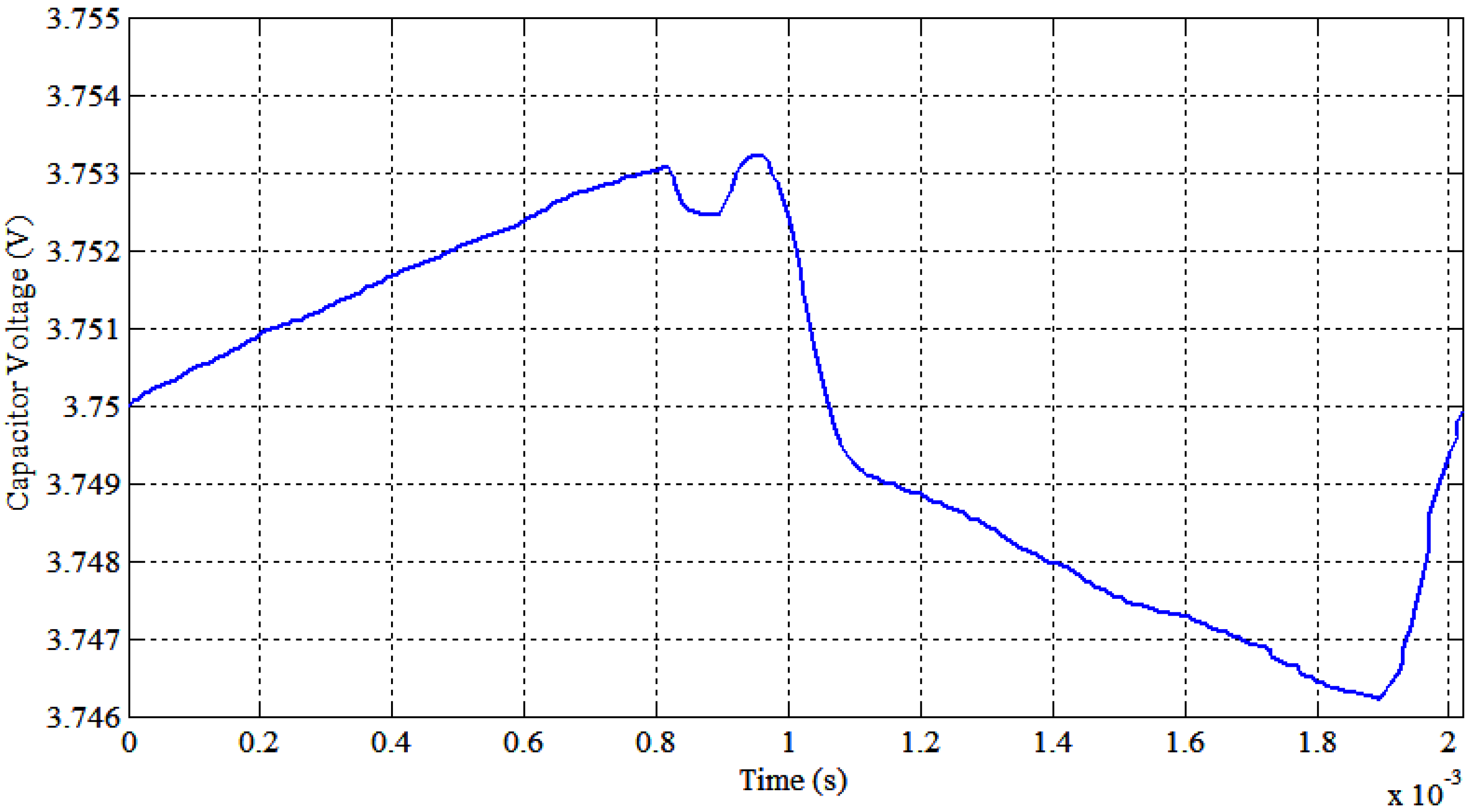7.
Some protective steps are necessary for experimental prototype implementation:
• When the balancing starts, and if the capacitor voltage is lower than the low charge cell voltage, the capacitor must be switched first to the lower voltage cell in order to prevent high capacitor charging current. Another solution to avoid high balancing current can be done by controlling the duty cycle, which can easily control the switched capacitor balancing current, as shown in Figure 15.
• The other limitation is the upper limit of the switching frequency, for reasons such as the manufacturer-recommended maximum operating frequency or the response time of the battery [27,28]. In other words, how many milliseconds are needed for the battery to reach its final voltage when applying a step current pulse. In addition the cells impedance will be increased at high frequency, leading to more losses and voltage drops.

#### 4.3. Proposed SSC Balancing Simulation

The previous example of four 12 Ah lithium-ion cells is simulated with the proposed SSC balancing system to validate the proposed control strategy. The cells initial SoCs are 87, 85, 78 and 76%, means that the higher SoC difference is 11% or 1.32 Ah.
Figure 19 illustrates the proposed single switched capacitor balancing topology simulation results. The figure presents the cells and the capacitor voltages, cells SoCs, cells currents, cells energies, cells energies summation and the auxiliary battery energy. As shown in Figure 19, the balancing time is nearly of 3.3 hours. The proposed SSC has high efficiency where during balancing it loss about 115 mWh. The proposed SSC balancing system reaches balance after 3.3 hours, compare with the normal SSC balancing system (3.4 hours). The system gives an efficiency of 99% (cells energies at end/cells energies at start).
Figure 19. Proposed single switched capacitor balancing simulation (top to bottom) the cells and the capacitor voltages, cells SoCs, currents, energies, summation of the cells energy and the auxiliary battery energy.
Figure 19. Proposed single switched capacitor balancing simulation (top to bottom) the cells and the capacitor voltages, cells SoCs, currents, energies, summation of the cells energy and the auxiliary battery energy.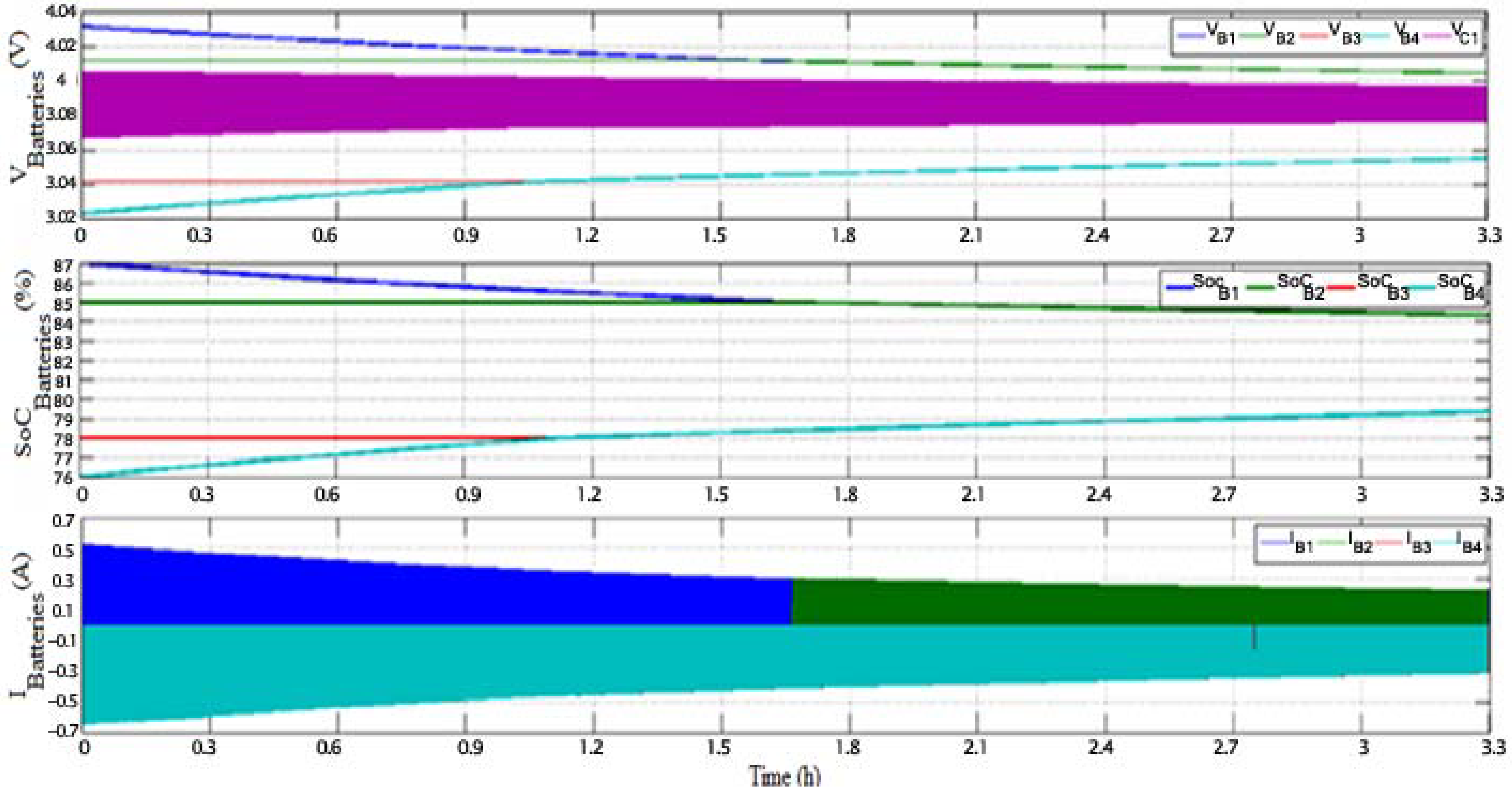## 5. Experimental Prototype and Experimental Results

This section describes the experimental implementation of the proposed SSC balancing system and the experimental results.

#### 5.1. Experimental Prototype

The prototype consists of two cells in series rated 2.3 Ah and 3.3 V. The SSC is used to achieve the system balancing with and without the proposed SSC balancing system.
The SSC balancing control circuits are simulated by using Proteus/ISIS® simulation program. The simulation using the ISIS program is the primary step to implement the experimental prototype. This simulation model can investigate the proposed control strategy by using the microcontroller. The ISIS program also allows to generate the circuit PCB.
The circuit elements marked by 1–16 will be mentioned in point 4.
The circuit of can be divided into four parts as shown in Figure 20. In addition, the experimental implementation of the SSC circuit is shown in Figure 21.
The circuit consists of four parts which are given as follows:
• The SSC main circuit including the cells connectors, the switches matrix, the capacitor and the boosting circuit.
• The first part of the SSC circuits contains the balancing capacitor series with current sensing resistor, main switches, protection fuses and the module’s cells’ connectors (see Figure 17).
• The bidirectional switches are implemented by using two anti-series “IRFB3806PBF” MOSFETs.
• The experimental tests are performed with 22 mF with maximum ESRs of 65 mΩ.
• The isolated boosting dc/dc converter “MEE3S1205SC” has an input/output voltage rating of 12/5 V and 0.6 A output current (3W).
• The switches isolation optocouplers and driving circuit.
• This circuit provides the isolation between the control circuit (microcontroller) and the power circuits (switches). The implementation of the switches isolation driver-circuit is performed by using TLP250 optocoupler, having a turn-on time of 0.5 μs.
• One converter for each bidirectional switch is implemented to insure the full isolation between the power circuit elements.
• Power supply circuit, sensors (such as current, voltage temperature sensors).
• The power circuit is supplied from the auxiliary battery through a 7812 voltage regulator. Then two MEE3S1215SC (12/15V, 3W) isolated converters are implemented to have ±15 V sources.
• The SSC balancing current is measured through of 10 mΩ resistor serially connected to the capacitor.
• The cells’ voltages are measured by using a differential method. A high precision operational amplifier “OPA4277PA”, with four op-amps inside, is used for this purpose and its outputs are connected directly to microcontroller’s ADC.
• Temperature sensor is illustrated using the thermometer “LM35DT”. They are four connectors to measure the four cells’ temperatures in the circuit.
• The fan power supply utilizes a 12 V (7812) voltage regulator. The fan speed is controlled by PWM output from the microcontroller.
• The microcontroller circuit
• The microcontroller “PIC18F4550”, which is used for controlling purpose technique with 20 MHz crystal.
• An additional high speed analog multiplexer/demultiplexer “74HC4051”, is used for future analog reading.
The SSC circuit which is shown in Figure 21 consists of:
• Microcontroller “PIC18F4550”;
• I2C interfacing connector;
• I2C interfacing isolation “ADUM1251”;
• Module power supply regulators “7812” circuit;
• Auxiliary battery input;
• Switches’ driver optocoupler isolation matrix “TLP250”;
• Switches’ driver isolated converter matrix “MEE3S1215SC”;
• Current sensing op-amps “LM324AN”;
• Voltage sensing op-amps “OPA4277PA”;
• Boosting converter “MEE3S1205SC”;
• Balancing current sensing resistor (10 mΩ);
• Capacitor connection points;
• Switches matrix;
• Protection fuses;
• Module’s cells’ connectors;
• Cells temperature sensors connectors;
• Analog multiplexer/demultiplexer “74HC4051”;
• Cooling fan control circuit and its connectors.
Figure 20. SSC control circuit global view.
Figure 20. SSC control circuit global view.Figure 21. SSC circuit PCB prototype.
Figure 21. SSC circuit PCB prototype.#### 5.2. Experimental Results

Experimental results are obtained from two tests, which are carried out on two series LFP cells rated 2.3 Ah and 3.3 V. In these tests, the cells are balanced by using the SSC balancing system without and with the proposed balancing technique. The two 2.3 Ah cells’ tests have been realized according to the following conditions:
• Two tests have been performed on these cells. The two cells started with voltages of 3.288 and 3.212 V (Vdiff. = 76 mV). The initial SoCs of these cells are around 35% and 11.3%, respectively (SoC difference is around 23.7% or 545 mAh);
• The two tests are performed with 22 mF (maximum ESRs of 65 mΩ) at switching frequency of 588 Hz (T = 1.7 ms);
• The cells and the capacitor voltages are measured by using Fluke “177” digital multimeter with an accuracy of ±0.09%;
• These tests are performed by using open-loop control. It means that the controller did not measure the cells’ voltages, it is directly shuttling the energy between the two cells;
• The time of tests is around 24 hours for the balancing between cells, where there is a rest of nearly 12 hours after the first 12 hours of balancing;
• First test (no. 1) is carried out without using the proposed balancing technique;
• Second test (no. 2) is carried out with using the proposed balancing technique. The test has fixed boosting pulses of 40 μs;
The results of test no. 1 is shown in Figure 22 and test no. 2 in Figure 23.
Figure 22. Experimental results of 2.3 Ah cells with normal SSC balancing.
Figure 22. Experimental results of 2.3 Ah cells with normal SSC balancing.Figure 23. Experimental results of 2.3 Ah cells with proposed SSC balancing.
Figure 23. Experimental results of 2.3 Ah cells with proposed SSC balancing.As mentioned before, at the starting of balancing the cells voltages were 3.288 and 3.212 V (Vdiff. = 76 mV) and the SoCs were 35% and 11.3% (SoC difference is 23.7% or 545 mAh) and the SoC summation was 46.3% (this is used as an indication to the total charges in the cells). Table 2 summarizes the previous experimental testing results. In addition, this table is followed by the description and the conclusions of the above tests’ Figures.
Table 2. Two 2.3 Ah cells balancing experimental testing results using SSC.
Table 2. Two 2.3 Ah cells balancing experimental testing results using SSC.
Test No.C (mF)F (Hz)Balancing time (h)OCV @ balanceSoC @ balanceNotes
Cell1 (V)Cell2 (V)Diff. (mV)Cell1 (%)Cell2 (%)Diff. (%)Sum. (%)
122588203.2513.247422.521.31.243.84 mV till 24 h
222588193.2533.252123.222.90.346.14 mV @ 14 h
From Table 2 it is easy to conclude the following:
• As can be seen in test no. 1: the minimum voltage difference, which can be obtained is 4 mV. This voltage is obtained after 20 hours and still constant (4 mV) and remains constant till 24 hours of test. In addition, this Li-ion flat voltage region gives SoC difference around 8% according to the voltage difference (4 mV).
• The proposed SSC’s control topology test no. 2: give the smallest voltage difference (1 mV) between the cells with the smallest balancing time (19 hours). This small voltage difference can overcome lithium iron phosphate batteries’ flat voltage balancing problems. The cells reach a voltage difference of 4 mV at 14 hours.
• Comparing the two tests with the same capacitor value and the switching frequency. For the same final voltage differences (4 mV) the proposed SSC balancing system (second test) reach it after 14 hours and the normal SSC balancing (first test) reach it after 20 hours. That means the proposed SSC balancing reduces the balancing time by 30%.

## 6. Conclusions

Cell balancing is a key task of the battery management system. It increases battery pack lifetime, the safety of the battery system, as well as optimizing the whole battery pack capacity. Shuttling capacitor battery balancing topologies (SC, DTSC, SSC and MSC) have been reviewed and simulated with the aid of MATLAB/Simulink®. A comparative study between these topologies has been performed including: simulation results, circuits’ description, implementations, balancing speed. The single switched capacitors balancing method has been discussed in detail.
A novel control strategy for the SSC balancing system has been proposed. The advantages of the proposed control strategy are: reducing the system size, cost as well as the balancing time. This is done by maximizing the energy transfer between the cell(s) and the capacitor. Furthermore, the SSC (or the shuttling capacitor) balancing with the Li-ion cells’ flat voltage problem has been solved.
The simulation and experimental results prove the validity of the extracted transferred energy function, as well as, the proposed technique to control the SSC balancing systems.
The proposed balancing system SSC gives good results during the system simulation and experimental tests. The proposed balancing system reduces the balancing time by 30%. Furthermore, it gives very small voltage difference (1 mV).

## Abbreviations

 BMS Battery management systems DTSC Double-tiered switched capacitor balancing EV Electric vehicle EPNGV Extended Partnership for a New Generation of Vehicles Li-ion Lithium-Ion Li-Po Lithium-Polymer MSC Modularized switched capacitor balancing PWM Pulse width modulation RUL Remaining useful life SC Switched capacitor balancing SoC State of charge (%) SoH State of health (%) SSC Single Switched Capacitor balancing

## References

1. Daowd, M.; Omar, N.; van den Bossche, P.; van Mierlo, J. A review of passive and active battery balancing based on MATLAB/Simulink. J. Int. Rev. Electr. Eng. 2011, 6, 2974–2989. [Google Scholar]
2. Daowd, M.; Omar, N.; van den Bossche, P.; van Mierlo, J. Passive and Active Battery Balancing Comparison based on MATLAB Simulation. In Proceedings of the IEEE Vehicle Power and Propulsion Conference (VPPC), West Chicago, IL, USA, 6–9 September 2011; pp. 1–7.
3. Jian, C.; Schofield, N.; Emadi, A. Battery Balancing Methods: A Comprehensive Review. In Proceedings of the IEEE Vehicle Power and Propulsion Conference (VPPC ’08), Harbin, China, 3–5 September 2008; pp. 1–6.
4. Kong, Z.-G.; Zhu, C.-B.; Lu, R.-G.; Cheng, S.-K. Comparison and Evaluation of Charge Equalization Technique for Series Connected Batteries. In Proceedings of the 37th IEEE Power Electronics Specialists Conference (PESC ’06), Jeju, South Korea, 18–22 June 2006; pp. 1–6.
5. Moore, S.W.; Schneider, P.J. A Review of Cell Equalization Methods for Lithium Ion and Lithium Polymer Battery Systems. In Proceedings of the SAE 2001 World Congress, Detroit, MI, USA, 5–8 March 2001.
6. Isaacson, M.J.; Hollandsworth, R.P.; Giampaoli, P.J.; Linkowsky, F.A.; Salim, A.; Teofilo, V.L. Advanced Lithium Ion Battery Charger. In Proceedings of the Fifteenth Annual Battery Conference on Applications and Advances, Long Beach, CA, USA, 11–14 January 2000; pp. 193–198.
7. Kutkut, N.H.; Divan, D.M. Dynamic Equalization Techniques for Series Battery Stacks. In Proceedings of the 18th International Telecommunications Energy Conference (INTELEC ’96), Boston, MA, USA, 6–10 October 1996; pp. 514–521.
8. Lindemark, B. Individual Cell Voltage Equalizers (ICE) for Reliable Battery Performance. In Proceedings of the 13th International Telecommunications Energy Conference (INTELEC ’91), Kyoto, Japan, 5–8 November 1991; pp. 196–201.
9. Stuart, T.A.; Wei, Z. Fast equalization for large lithium ion batteries. IEEE Aerosp. Electron. Syst. Mag. 2009, 24, 27–31. [Google Scholar] [CrossRef]
10. Kimball, J.W.; Kuhn, B.T.; Krein, P.T. Increased Performance of Battery Packs by Active Equalization. In Proceedings of the IEEE Vehicle Power and Propulsion Conference (VPPC ’07), Arlington, TX, USA, 9–12 September 2007; pp. 323–327.
11. Kobzev, G.A. Switched-capacitor Systems for Battery Equalization. In Proceedings of Modern Techniques and Technology, MTT 2000, 6th International Scientific and Practical Conference of Students, Post-graduates and Young Scientists, Tomsk, Russia, 28 February–3 March 2000; pp. 57–59.
12. West, S.; Krein, P.T. Equalization of Valve-regulated Lead-acid Batteries: Issues and Life Test Results. In Proceedings of the Twenty-second International Telecommunications Energy Conference (INTELEC ’00), Dresden, Germany, 7–10 May 2000; pp. 439–446.
13. Pascual, C.; Krein, P.T. Switched Capacitor System for Automatic Series Battery Equalization. In Proceedings of the Twelfth Annual Applied Power Electronics Conference and Exposition (APEC ’97), Atlanta, Georgia, 23–27 February 1997; Volume 842, pp. 848–854.
14. Baughman, A.C.; Ferdowsi, M. Double-tiered switched-capacitor battery charge equalization technique. IEEE Trans. Ind. Electron. 2008, 55, 2277–2285. [Google Scholar] [CrossRef]
15. Baughman, A.; Ferdowsi, M. Analysis of the Double-Tiered Three-Battery Switched Capacitor Battery Balancing System. In Proceedings of the IEEE Vehicle Power and Propulsion Conference (VPPC ’06), Windsor, UK, 6–8 September 2006; pp. 1–6.
16. Baughman, A.; Ferdowsi, M. Double-tiered Capacitive Shuttling Method for Balancing Series-connected Batteries. In Proceedings of the 2005 IEEE Conference on Vehicle Power and Propulsion, Chicago, IL, USA, 7–9 September 2005; pp. 109–113.
17. Speltino, C.; Stefanopoulou, A.; Fiengo, G. Cell Equalization in Battery Stacks through State of Charge Estimation Polling. In Proceedings of the American Control Conference (ACC’10), Baltimore, MD, USA, 30 June–2 July 2010; pp. 5050–5055.
18. Daowd, M.; Omar, N.; van den Bossche, P.; van Mierlo, J. Capacitor Based Battery Balancing System. In Proceedings of the 26th Hybrid and Fuel Cell Electric Vehicle Symposium (EVS-26), Los Angeles, CA, USA, 6–9 May 2012; pp. 1–9.
19. Park, H.S.; Kim, C.H.; Park, K.B.; Moon, G.W.; Lee, J.H. Design of a charge equalizer based on battery modularization. IEEE Trans. Veh. Technol. 2009, 58, 3216–3223. [Google Scholar] [CrossRef]
20. Al Sakka, M.; Gualous, H.; van Mierlo, J.; Culcu, H. Thermal modeling and heat management of supercapacitor modules for vehicle applications. J. Power Sources 2009, 194, 581–587. [Google Scholar]
21. Yonghua, C.; Joeri, V.M.; Lataire, P.; Buechel, M.; Knorr, R.; Gallay, R. Method of Identifying Voltage Difference of Super Capacitors and Principle of Voltage Balancing. In Proceedings of the 2007 European Conference on Power Electronics and Applications, Aalborg, Denmark, 2–5 September 2007; pp. 1–10.
22. Daowd, M.; Omar, N.; van den Bossche, P.; van Mierlo, J. A comparative study of battery models parameter estimation. J. Int. Rev. Electr. Eng. 2012, 7, 4915–4924. [Google Scholar]
23. Daowd, M.; Omar, N.; Verbrugge, B.; van den Bossche, P.; van Mierlo, J. Battery Models Parameter Estimation based on MATLAB/Simulink. In Proceedings of the 25th Electric Vehicle Symposium (EVS-25), Shenzhen, China, 5–9 November 2010.
24. Daowd, M.; Omar, N.; van den Bossche, P.; van Mierlo, J. Extended PNGV battery model for electric and hybrid vehicles. J. Int. Rev. Electr. Eng. 2011, 6, 1264–1278. [Google Scholar]
25. Kimball, J.W.; Krein, P.T. Analysis and Design of Switched Capacitor Converters. In Proceedings of the Twentieth Annual IEEE Applied Power Electronics Conference and Exposition (APEC’05), Austin, TX, USA, 6–10 March 2005; Volume 1473, pp. 1473–1477.
26. Ben-Yaakov, S.; Evzelman, M. Generic and unified model of Switched Capacitor Converters. In Proceedings of the IEEE Energy Conversion Congress and Exposition (ECCE’09), San Jose, CA, USA, 20–24 September 2009; pp. 3501–3508.
27. Jossen, A. Fundamentals of battery dynamics. J. Power Sources 2006, 154, 530–538. [Google Scholar] [CrossRef]
28. Ke, Z.; Nawrocki, S.; Renxiang, W.; Jin, W. High Current Battery Impedance Testing for Power Electronics Circuit Design. In Proceedings of the Vehicle Power and Propulsion Conference, (VPPC ’09), Dearborn, MI, USA, 7–10 September 2009; pp. 531–535.
Back to TopTop